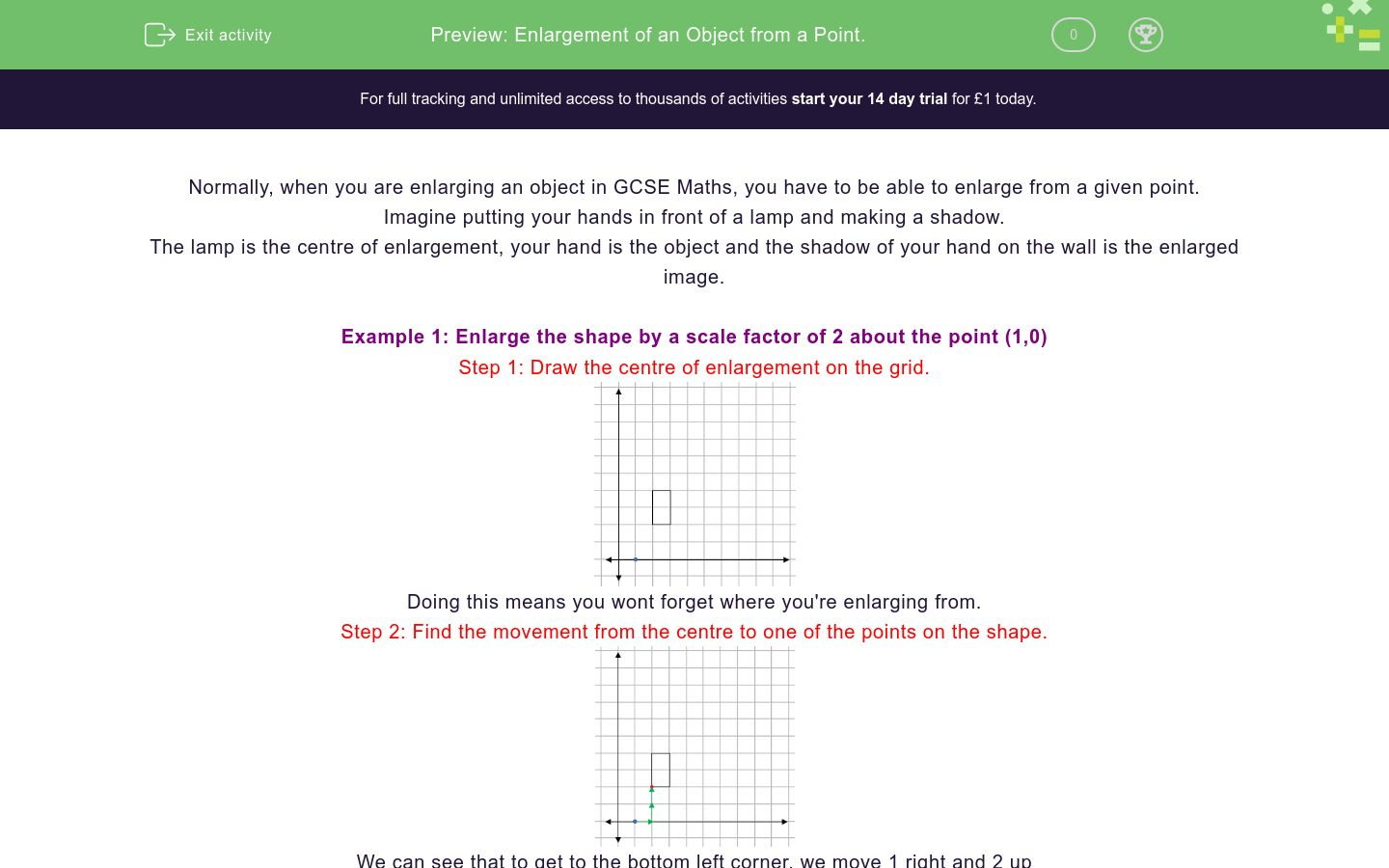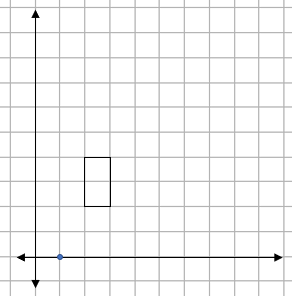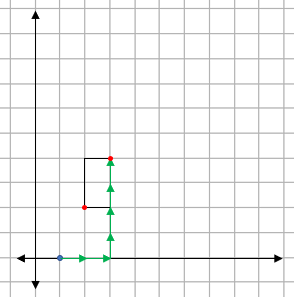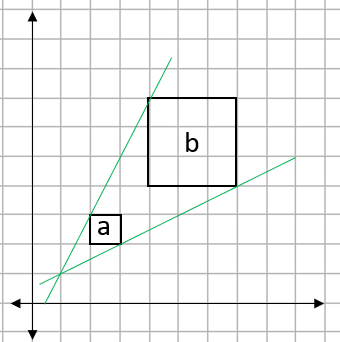# Enlargement of an Object from a Point.

In this worksheet, students practise enlarging a shape from a given point.Key stage:  KS 4

GCSE Subjects:   Maths

GCSE Boards:   AQA, Eduqas, Pearson Edexcel, OCR

Curriculum topic:   Geometry and Measures, Congruence and Similarity

Curriculum subtopic:   Properties and Constructions, Similarity

Difficulty level:### QUESTION 1 of 10

Normally, when you are enlarging an object in GCSE Maths, you have to be able to enlarge from a given point.

Imagine putting your hands in front of a lamp and making a shadow.

The lamp is the centre of enlargement, your hand is the object and the shadow of your hand on the wall is the enlarged image.

Example 1: Enlarge the shape by a scale factor of 2 about the point (1,0)

Step 1: Draw the centre of enlargement on the grid.Doing this means you wont forget where you're enlarging from.

Step 2: Find the movement from the centre to one of the points on the shape.We can see that to get to the bottom left corner, we move 1 right and 2 up

Step 3: Multiply this movement by the Scale Factor.

Our scale factor is 2, so this movement doubles to 2 right and 4 up.

Step 4: Plot this point on the diagram.

When you are plotting this point, it is vital that you count from the centre of enlargement.Step 5: Repeat steps 2 - 4 for the other corners of the shape.Step 6: Draw the enlarged shape and label the image and enlargement.Example 2: Shape A is enlarged to shape B. Find the scale factor and centre of enlargement.Finding the Scale Factor.

All we have to do here is to look at one of the pairs of sides. If we use the top of each shape, shape A is 1cm long and shape B is 3 cm long.

This gives a scale factor of 3.

Finding the centre of enlargement.

To find this, we join up two pairs of matching corners with striaght lines and see where they join up.We can see here that the two lines join up at the point (1,1). This is the centre of enlargement.

To describe an enlargement, we need to have...

Rectangle ABCD is enlarged by SF 2 around the point (0,0).Find the coordinates of a'

Rectangle ABCD is enlarged by SF 2 around the point (0,0).Find the coordinates of d'

Rectangle ABCD is enlarged by SF 3 around the point (1,0).Find the coordinates of b'

Rectangle ABCD is enlarged by SF 3 around the point (1,0).Find the coordinates of c'

Shape a is enlarged around the point (0,0). What is the SF of the enlargement?Shape a is enlarged to shape a'. Find the SF and centre of the enlargement.In an enlargement we have two shapes labelled a and a'...

The triangle abc is enlarged with a SF of  2 about the point (2,2).What are the coordinates of a',b' and c'

 Coordinate a' b' c'

The rectangee abcd is enlarged with a SF of  3 about the point (3,0).What are the coordinates of a',b',c' and d'

 Coordinate a' b' c' d'
• Question 1

To describe an enlargement, we need to have...

EDDIE SAYS
To enlarge from a point, we need to know the change of size and the point that we enlarge from.
• Question 2

Rectangle ABCD is enlarged by SF 2 around the point (0,0).Find the coordinates of a'

(4,4)
EDDIE SAYS
To get from the centre (0,0) to point A is a movement of 2 right and 2 up. If we double this (SF 2), we get a movement of 4 right and 4 up. We then do this movement from the centre to get the point a\'.
• Question 3

Rectangle ABCD is enlarged by SF 2 around the point (0,0).Find the coordinates of d'

(4,6)
EDDIE SAYS
To get from the centre (0,0) to point D is a movement of 2 right and 3 up. If we double this (SF 2), we get a movement of 4 right and 6 up. We then do this movement from the centre to get the point d'.
• Question 4

Rectangle ABCD is enlarged by SF 3 around the point (1,0).Find the coordinates of b'

(10,6)
EDDIE SAYS
To get from the centre (1,0) to point B is a movement of 3 right and 2 up. If we triple this (SF 3), we get a movement of 9 right and 6 up. We then do this movement from the centre to get the point b'.
• Question 5

Rectangle ABCD is enlarged by SF 3 around the point (1,0).Find the coordinates of c'

(10,9)
EDDIE SAYS
To get from the centre (1,0) to point C is a movement of 3 right and 3 up. If we triple this (SF 3), we get a movement of 9 right and 9 up. We then do this movement from the centre to get the point b'.
• Question 6

Shape a is enlarged around the point (0,0). What is the SF of the enlargement?2
EDDIE SAYS
If we count the length of the sides a and a', we see that a' is twice the length of a.
• Question 7

Shape a is enlarged to shape a'. Find the SF and centre of the enlargement.EDDIE SAYS
Comparing the sides shows that a' is three times longer than a Drawing lines back through the corners will give the centre of enlargement.
• Question 8

In an enlargement we have two shapes labelled a and a'...

EDDIE SAYS
We always use this dash notation to indicate which one is the transformed shape. If you see anything with a dash, its the result.
• Question 9

The triangle abc is enlarged with a SF of  2 about the point (2,2).What are the coordinates of a',b' and c'

 Coordinate a' b' c'
EDDIE SAYS
Does it matter that the centre of enlargement is in the middle of the shape? Not at all, we do it in the same way. For example, to get the coordinate of c\'. We find the movement from our centre (2,2) to the point C - This is 1 square up Doubling this (SF2) gives a movement of 2 up from the centre. This gives the coordinate of c\' as (2,4)
• Question 10

The rectangee abcd is enlarged with a SF of  3 about the point (3,0).What are the coordinates of a',b',c' and d'

 Coordinate a' b' c' d'
EDDIE SAYS
Does it matter that the shape goes over the y - axis? Not at all, we do it in the same way. For example, to get the coordinate of a'. We find the movement from our centre (0,3) to the point 1 - This is 2 left and 1 square up Doubling this (SF2) gives a movement of 6 left and 3 up from the centre This gives the coordinate of a' as (-3,6)
---- OR ----

Sign up for a £1 trial so you can track and measure your child's progress on this activity.

### What is EdPlace?

We're your National Curriculum aligned online education content provider helping each child succeed in English, maths and science from year 1 to GCSE. With an EdPlace account you’ll be able to track and measure progress, helping each child achieve their best. We build confidence and attainment by personalising each child’s learning at a level that suits them.

Get started